# GED Math

The math portion of the GED test is what concerns most people.We believe that with a little studying and some guidance you will pass the GED math section.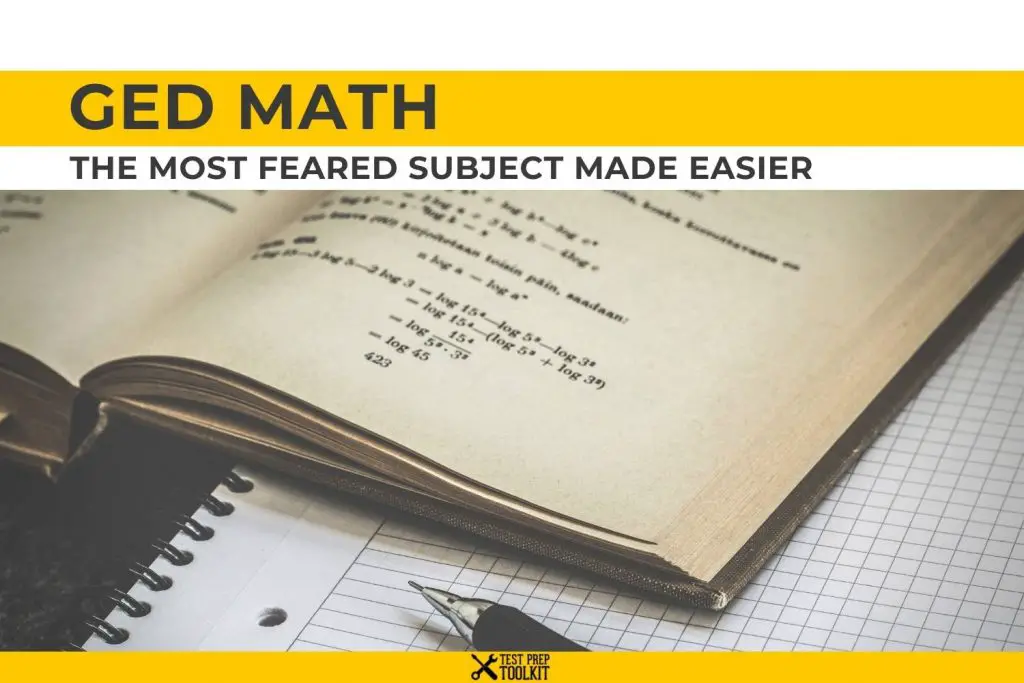DISCLOSURE: This post may contain affiliate links, meaning when you click the links and make a purchase, we receive a commission.

## GED Math: The Most Feared Subject made Easier.

The math portion of the GED test is what concerns most people. Sadly, it even stops many from taking the GED test in the first place. But rest assured! We believe that with a little studying and some guidance to student, you will pass the GED math section with flying colors.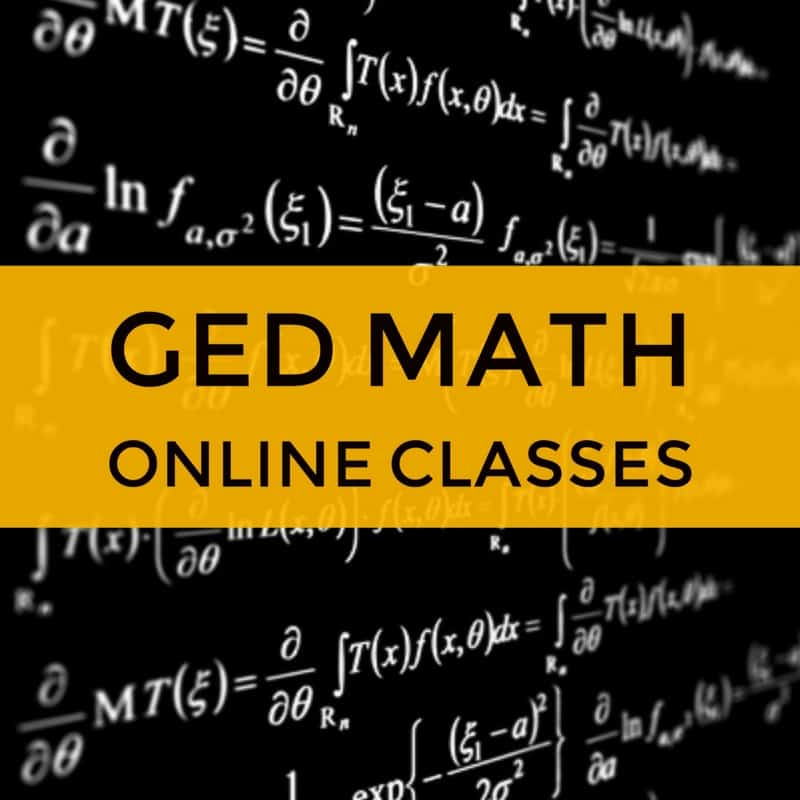GED Math Blueprint Course45 in-depth videos lessons + 3 Practice Tests (We also include 100s of Practice Questions for FREE)

Watch our Online GED Math Videos covering all topics you will face during the GED Math test

### What is the GED Math Test Like?

**Please note that the time allotted for the GED math has been increased to 115 minutes.

Are you overwhelmed just thinking about the GED math test? Has it been a few years since you’ve studied math? Was math your least favorite subject in school? It’s okay, you certainly are not alone.

## What Does the GED Math Test Cover?

The GED® Math test cover topics like:

• Number operations & number sense = 20-30%
• Measurement & geometry = 20-30%
• Data analysis, statistics, & probability = 20-30%
• Algebra, functions, & patterns = 20-30%
The GED Math test has 46 questions. You need to finish the test in 115 minutes or less. You are required to get a correct score of 145 to pass this section. A calculator and formula and symbols sheet will be provided.

The test is divided into two parts:

First part: 5 test questions
Calculator is not allowed here.

Second part: 41 test questions
You will be allowed to use the calculator here.

We have Practice Test that you can use: Free GED Social Studies Practice Test

The GED Math Test covers materials, like:

• Number operations and number sense
• Measurement and geometry
• Data analysis, statistics, and probability
• Algebra, functions, and patterns

Test Prep Tool Kit designed practice tests and online classes to take the fear out of GED math. We understand how GED math can feel downright scary. Math can intimidate you If you haven’t studied the subject for years. It’s even scarier if you have always found math difficult.

Our GED math practice tests contain 25 practice questions that you can study at your own pace. Answers can be quickly revealed after each question. Our  GED math online have 25 classes, with 3 to 7-minute videos on math topics found in the actual GED math test. Get our GED Study Guide for tips on how to prepare for GED math test and other subjects.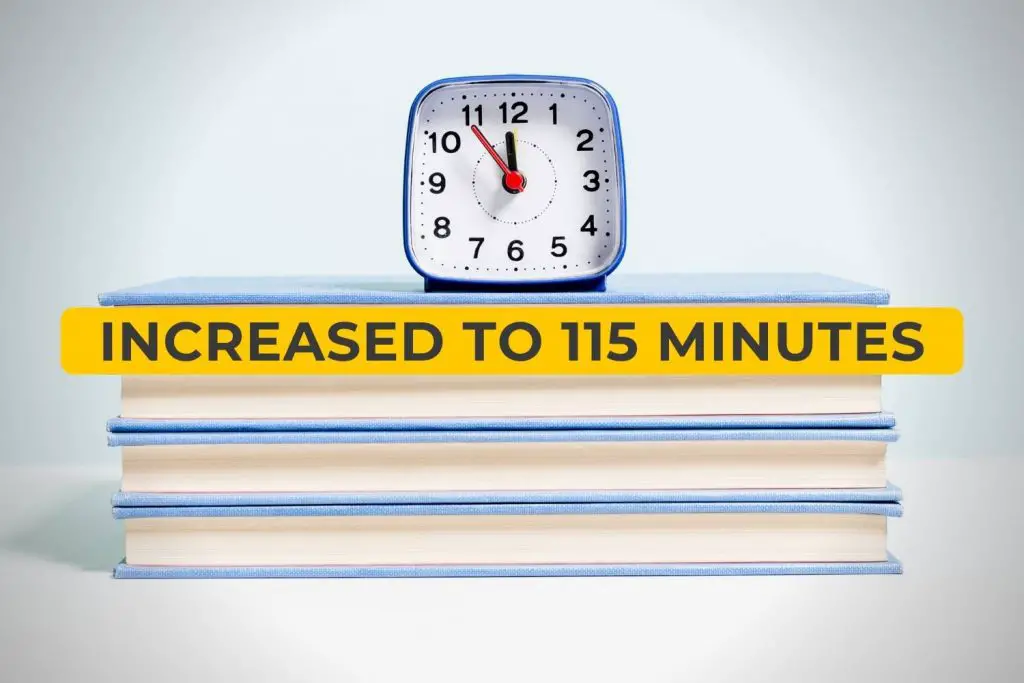Start reviewing with our helpful contents: GED Science Classes Online

# Tips for Success in the GED Math Test

GED math is the most feared subject in this high school certification test. Math anxiety is real. The Journal of Neuroscience defines it as stress or anxiety that one feels when answering math problems. Fear of math may affect your chance to succeed in life. Fear of math may prevent the student from passing the GED math test.

Are you afraid of math? A Stanford education expert says the fear may have come from the pressure to learn it fast. Timed tests may have caused you to fail before. Memorizing methods instead of understanding their application in numbers may have prevented you from learning the right way.

The good news is, you can get rid of math anxiety. You can change the way you feel about math by tutoring yourself. Tutoring yourself is possible. You can do this by taking GED practice tests or GED online classes that we have here at Test Prep Tool Kit.

• Some questions on the GED® math test will be directly related to a given number line, chart, graph, word problem or other types of statistical data.

We have GED Math Video Lessons

• Questions on the GED® Math Test will require you to use common formulas. Use the formula sheet as your guide.

*TIP: Be familiar with these formulas so won’t waste time looking them up. If you have these formulas memorized, you will be able to simply plug in the information provided.

Q: Will a calculator be allowed on the test?
A: Yes. You will have access to an on-screen calculator, which is a Texas Instruments TI-30XS Multi-View calculator. Or, you may bring your Texas Instruments TI-30XS Multi-View calculator to the test. It must be stored in designated areas and you will be notified when you are allowed to gain access to it.

Calculators aren’t allowed in the part of the test where arithmetic skills and basic number sense are involved. This is the first part of the test.

Q: What type of content will be on the GED® Math Test?
A: There are two content areas that will be tested on the GED test®: Quantitative and Algebraic. Test-takers should be familiar with:

1. Quantitative Problem Solving (makes up 45% of the test)
2. Algebraic Problem Solving(makes up 55% of the test)

We have Practice Test that you can use: GED® Reasoning Through Language Arts Practice Tests

Q: What are Quantitative Problem Solving Questions?
A: These types of questions revolve around Number Operations and Geometric Thinking.

Q: What types of questions will be asked on the GED® Math Test?
A: There are five types of questions on the exam:

1. Multiple Choice
2. Fill-in-the-blank
3. Hot-spot
4. Drag and drop
5. Drop-down

Q: What level of Mathematical Reasoning will the GED® Math Test measure?
A: The test will check if your math skills are ready for college and for the workforce.

Q: How many questions are on the GED® Math Test?
A: The GED math test has 46 questions.

our GED® Social Studies Prep Guide

## Common Formulas and Terms to Know for the GED® Math Test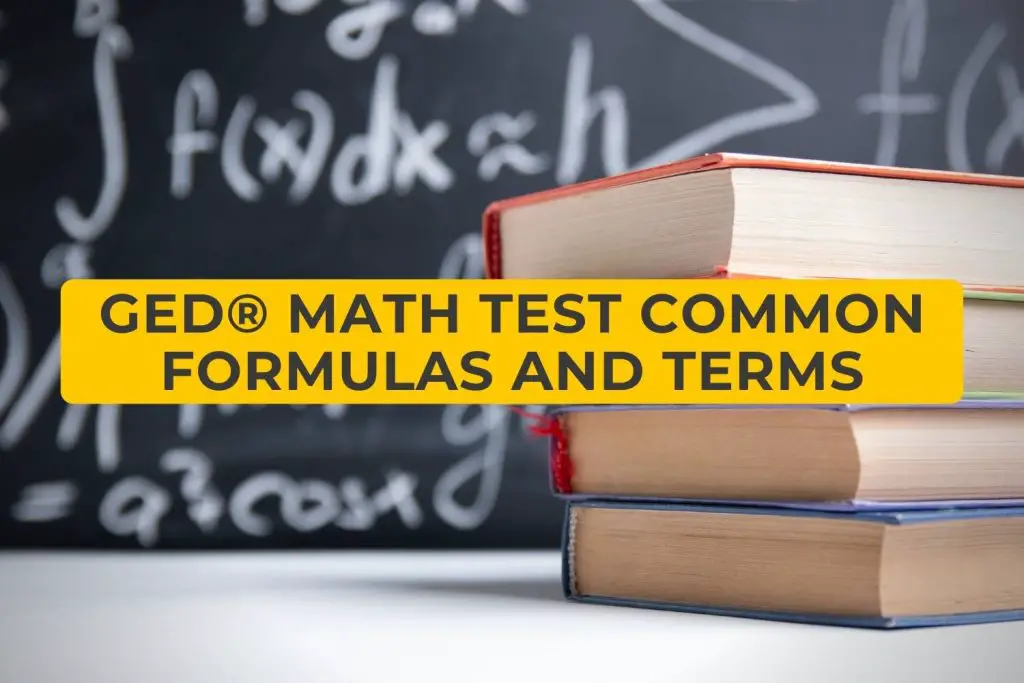Be familiar with these common formulas before you take the GED® math test.

Perimeter: the distance around a shape.

• To find the perimeter of any shape add all lengths and widths together.

Area: The space that fills a shape

• Area of a square: side(side)
• Area of a rectangle: Length (width)
• Parallelogram: Base (height)
• Triangle: 1/2 (base)(height)

Pythagorean Formula

•## Complete GED® Math Study Guide Checklist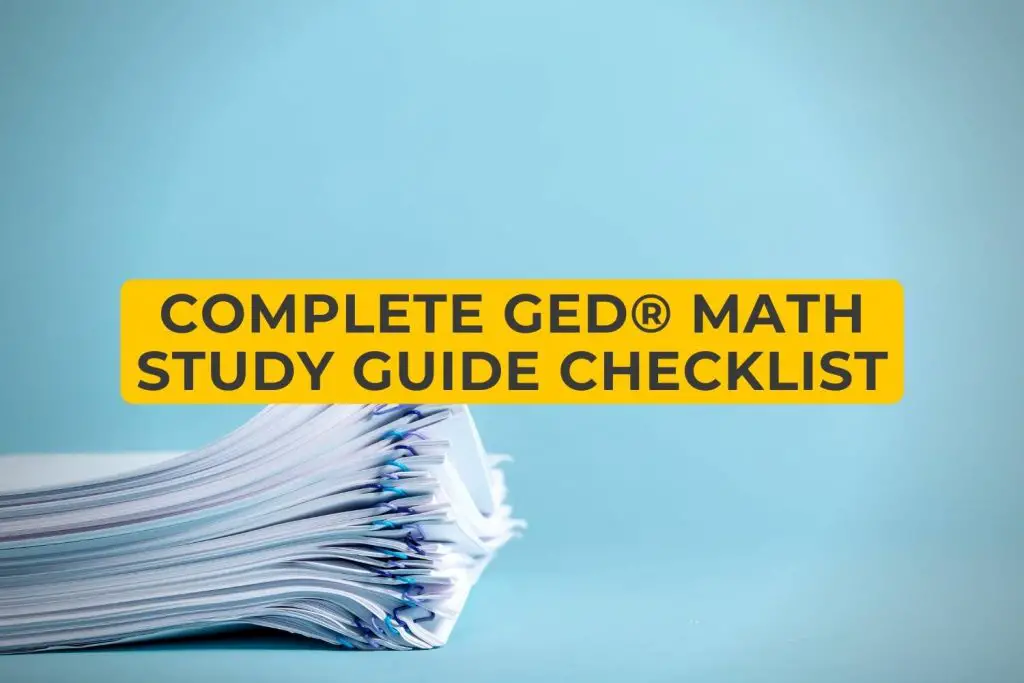You should know WHAT concepts you will be expected to know for each test area. Below is a checklist of all the math concepts and topics. Under each topic are questions that will guide you through your studying. It should be your goal to know each of these concepts prior to the exam day.

Use this list as you study. Once you learn a concept, you should move on to the next. Once you’re confident with these study topics, head over to our Free Online GED® Math Practice Test.

NUMBER SENSE: Rational Numbers, Absolute Value, Multiples, Factors, and Exponents

• Can I order Fractions and Decimals on a Number Line?
• Can I use number properties, like multiples and factors? And can I use greatest common factors, least common factors, and/or distributive property to rewrite numeric expressions?
• Can I use the rules of exponents with numerical expressions, with rational exponents to write equivalent expressions, with rational exponents?
• Number lines: Can I determine absolute values or rational numbers on a number line? Can I determine the distance between two numbers on a number line? Can I use their absolute value in determining their distance?

EXPONENTS, ROOTS of RATIONAL NUMBERS, FRACTIONS and DECIMALS: Adding, subtracting, multiplying and dividing.

• Can I add, multiply, subtract and divide rational numbers?
• Can I compute and write numerical expressions with squares and square roots of positive, rational numbers?
• Can I determine if and when a numerical expression is undefined?
• Can I figure out practical real-world problems, and one-step or multi-step arithmetic problems involving addition, subtraction, division and/or multiplication with rational numbers, including those involving scientific notation?

More review materials HERE

RATIOS, PERCENTS and SCALE FACTORS

• Can I compute unit rates?
• Can I use scale factors to determine the magnitude of size change? Can I convert between actual drawings and scale drawings?
• Can I solve multi-step, arithmetic, real-world problems using ratios or proportions including those that require converting units of measure?
• Can I figure out two-step, arithmetic, real world problems involving percents?

DIMENSIONS, PERIMETER, CIRCUMFERENCE, and AREA: two-dimensional figures.

• Can I compute area and perimeter of triangles and rectangles? Can I determine side lengths or a set of triangles and rectangles when given area or perimeter?
• Can I compute the area and circumference of circles? Can I determine radius or diameter when given an area or circumference?
• Can I compute the perimeter of a polygon? Can I compare the area of a polygon when given a geometric formula? Can I determine side lengths of polygons when given perimeter or area?
• Can I compute perimeter and area of 2-D composite geometric figures, which could include circles?
• Can I use the Pythagorean Theorem to determine unknown side lengths in a right triangle?

We have GED® Reasoning Through Language Arts Guide

Check our Math Blueprint Video Course covering every possible topic for GED Math. It includes +100 videos, +2000 practice questions and loads of information.

DIMENSIONS, SURFACE AREA, and VOLUME: Three-Dimensional Figures

• Can I compute volume and surface area or rectangular prisms when given geometric formulas? Is there a solution for side lengths or height when given volume or surface area?
• Can I compute volume and surface area of cylinders when given geometric formulas?
• Can I solve for height, radius or diameter when given volume or surface area?
• Can I compute volume and surface area of right prisms when given geometric formulas? Can I figure out for side lengths or height when given volume or surface area?
• Can I compute volume and surface area of right pyramids and cones when given geometric formulas? Can I figure out for side lengths, height, radius, or diameter when given volume or surface area?
• Can I compute volume and surface area of spheres when given geometric formulas? Can I figure out for radius or diameter when given the surface are?
• Can I compute surface area and volume of composite 3-D geometric figures, given geometric formulas?

INTERPRET and CREATE DATA ANALYSIS

• Can I represent, display, and interpret categorical data in bar graphs?
• Can I represent, display, and interpret data involving tone variable plots on the real number line including dot plots, histograms and box plots?
• Can I represent, display, and interpret data involving two variables in tables and the coordinate plane including scatter plots and graphs?

MEAN, MEDIAN, MODE and WEIGHTED AVERAGE: Calculate and Apply

• Can I calculate the mean, median and range?
• Can I calculate a missing data value, given the average and all the missing data values but one? As well as compute the average, given the frequency counts of all the data values, and calculating a weighted average?

COUNTING TECHNIQUES and PROBABILITIES

• Can I use counting techniques to figure out problems and determine combinations and permutations?
• Can I determine the probability of simple and compound events?

More helpful tips on our website: GED® Social Studies Prep Guide

EXPRESSIONS and POLYNOMIALS: Write, evaluate, and, compute.

• Can I add, subtract, factor, multiply and expand linear expressions with rational coefficients?
• Can I evaluate linear expressions by substituting integers for unknown quantities?
• Can I write linear expressions by substituting integers for unknown quantities?
• Can I write linear expressions as part of word-to-symbol translations or represent common settings?
• And I add subtract, multiply polynomials, including multiplying two binomials, or divide factorable polynomials?
• Can I evaluate polynomial expressions by substituting integers for unknown quantities?
• Can I factor polynomial expressions?
• Can I write polynomials expressions as part of word-to-symbol translations or represent common settings?
• Can I add, subtract, multiply and divide rational expressions?
• Can I evaluate rational expressions by substituting integers for unknown quantities?
• Can I write rational expressions as part of word-to-symbol translations or represent common settings?

LINEAR EQUATIONS: Write, manipulate, and Find out.

• Can I solve one-variable linear equations with rational number coefficients?
• Can I solve real-world problems involving linear equations?
• Can I write one-variable linear equations to represent context?
• Can I solve a system of two simultaneous linear equations by graphing, substitution, or linear combinations? Can I solve a set of real-world problems leading to the system of linear equations?

LINEAR INEQUALITIES: Write, Manipulate, Solve and Graph

• Can I figure out a set of linear inequalities of one variable with rational number coefficients?
• Can I solve a set of real-world problems involving inequalities?
• Can I write a set of linear inequalities in one variable to represent context?

More review materials here: Question 1 | GED Math Practice Test 3

QUADRATIC EQUATIONS: Write, Manipulate, and Find out.

• Can I solve a set of quadratic equations in one variable with rational coefficients and real solutions using appropriate methods?
• Can I write one-variable quadratic equations to represent a specific context?

GRAPHS AND FUNCTIONS: Connect and Interpret

• Can I locate given points on the coordinate plane?
• Can I determine the slope of a line when given a graph, equation, or table?
• Can I interpret unit rate as the slope of a proportional relationship?
• Can I graph two-variable linear equations?

For a function that models a linear or nonlinear relations between two quantities:

• Can I interpret key features of graphs and tables in terms of quantities?
• Can I sketch graphs showing key features of graphs and tables in terms of quantities,
• Can I sketch graphs showing key features given a verbal description of relations?

COORDINATES, LINES, and EQUATIONS

• Can I write the equation of a line with a given slope through a given point?
• Can I write an equation of a line passing through two given distinct points?
• Can I use the slope to identify parallel and perpendicular lines and to solve geometric problems?

FUNCTIONS: Compare, Represent, and Evaluate

• Can I compare two different proportional relationships represented in different ways?
• Can I represent or identify a function in a table of the graph as having exactly one output for each input?
• Can I evaluate linear and quadratic functions for values when they are represented with function notations?
• Can I compare properties of two linear and quadratic functions when they are represented in different ways?

### Now you have a solid plan for the GED math test.

Start studying now. Begin with our GED practice test for math. Then head on to our online classes. Our GED math online classes cover all the topics above.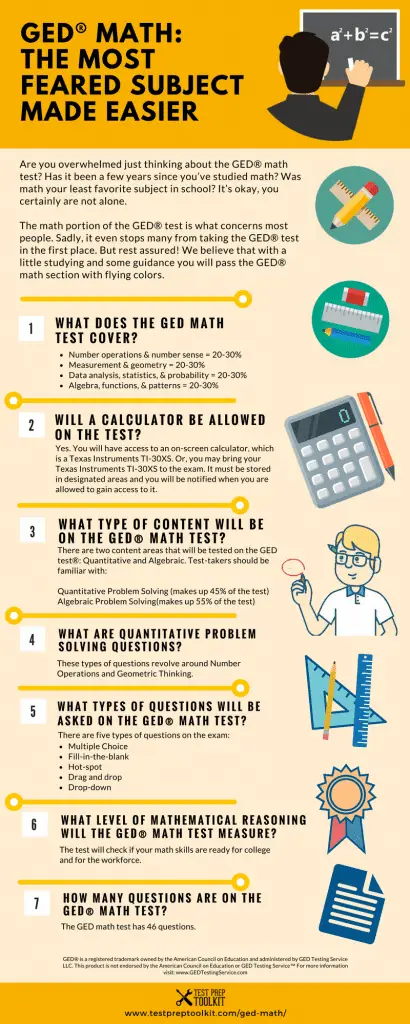Related Topics:

## Save Up To 20% Magoosh Test Prep

We’ve partnered with Magoosh Test Prep to get you EXCLUSIVE DISCOUNTS on a huge selection of test prep courses. We’ve seen a lot of students go through their programs and come out with a much higher test score than they otherwise would have.